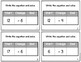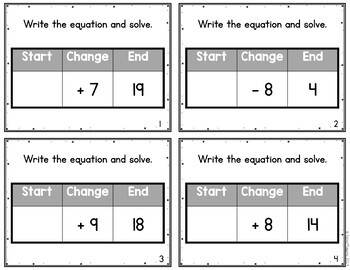# Start Change End Addition & Subtraction within 20 Problem Types Task CardsSubject
Resource Type
File Type

PDF

Standards
• Product Description
• StandardsNEW

When building an understanding of addition and subtraction it's important to teach the different problem types. These Start Change End equation table task cards have students recognizing the operations and using their knowledge of the inverse operations to solve for the missing number. These 9 task card sets give students practice with both addition and subtraction with 20, perfect for first grade math centers!

WHAT'S INCLUDED:

3 sets of addition task cards (start unknown, change unknown, end unknown)

• 3 sets of subtraction task cards (start unknown, change unknown, end unknown)

• 3 sets of mixed operations task cards (start unknown, change unknown, end unknown)

• 3 recording sheet options (open template, start change end tables, tens frames)

• covers for task card storage

Each task card set includes 24 different cards. Each card features a start-change-end table with one part unknown. Recording sheet options are included, and are the perfect differentiation tool. One sheet features tens frames so students have a concrete tool to solve the equation. Another sheet includes the start-change-end table for students to write the pieces before solving. The other option is a blank table for students to use the space for the strategy that works best for them. This tool is perfect for first grade math centers all year long!

YOU MAY ALSO LIKE Addition & Subtraction to 20 Start Change End Equation Table Task Cards

CLICK HERE to follow me and be notified of future products as soon as they are posted.

_______________________________________________________________

You may not redistribute, edit, sell, or otherwise post this product on the internet. You may, however, post a link for others to purchase themselves.

Determine the unknown whole number in an addition or subtraction equation relating three whole numbers. For example, determine the unknown number that makes the equation true in each of the equations 8 + ? = 11, 5 = ▯ - 3, 6 + 6 = ▯.
Add and subtract within 20, demonstrating fluency for addition and subtraction within 10. Use strategies such as counting on; making ten (e.g., 8 + 6 = 8 + 2 + 4 = 10 + 4 = 14); decomposing a number leading to a ten (e.g., 13 - 4 = 13 - 3 - 1 = 10 - 1 = 9); using the relationship between addition and subtraction (e.g., knowing that 8 + 4 = 12, one knows 12 - 8 = 4); and creating equivalent but easier or known sums (e.g., adding 6 + 7 by creating the known equivalent 6 + 6 + 1 = 12 + 1 = 13).
Understand subtraction as an unknown-addend problem. For example, subtract 10 – 8 by finding the number that makes 10 when added to 8.
Apply properties of operations as strategies to add and subtract. If 8 + 3 = 11 is known, then 3 + 8 = 11 is also known. (Commutative property of addition.) To add 2 + 6 + 4, the second two numbers can be added to make a ten, so 2 + 6 + 4 = 2 + 10 = 12. (Associative property of addition.)
Use addition and subtraction within 20 to solve word problems involving situations of adding to, taking from, putting together, taking apart, and comparing, with unknowns in all positions, e.g., by using objects, drawings, and equations with a symbol for the unknown number to represent the problem.
Total Pages
Included
Teaching Duration
N/A
Report this Resource to TpT
Reported resources will be reviewed by our team. Report this resource to let us know if this resource violates TpT’s content guidelines.